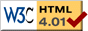# Moveable Double Pendulum

previous next
• Sim
• Graph
• Time Graph
• Multi Graph

Physics-based simulation of a double pendulum whose support point is moveable.

Click near the support point to drag it with your mouse. You can also reposition the pendulum masses. You can also change gravity, damping (friction), and various other parameters.

Experiments to try:

• Try dragging the anchor point by clicking near it with your mouse. Whip it around quickly back and forth -- does the pendulum behave as you would expect?
• Turn on "show energy". When is kinetic energy high? When is potential energy high?
• Set damping to zero, get the pendulum moving rapidly, and turn on "show energy". You will notice the total energy decreasing slightly over time, although it should stay constant. Now try slowing down time by a factor of 3 or 4 (which gives more accurate computation). Is the energy still decreasing? What's going on?

The math for this simulation is similar to the regular Double Pendulum with immobile support point, except there are extra terms carried forward into the equations for the support point velocity and acceleration. This is similar to the development of equations shown for the Moveable Pendulum. This movement of the support point is an input to the equations of motion, which means that the support point is not affected by the motion of the double pendulum. We assume the anchor/support point is so massive compared to the pendulum that it is not affected by the motion of the pendulums.

Also available are: open source code, documentation and a simple-compiled version which is more customizable.

previous next